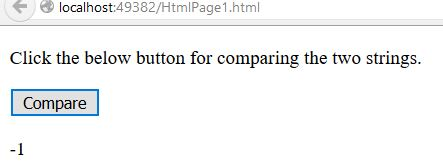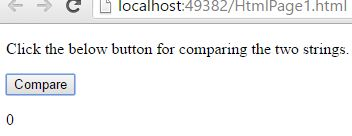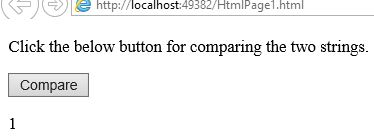# LocaleCompare() String in JavaScript

### Locale Compare

Local Compare is a string method {`localCompare()` method} in JavaScript, which is used to compare two strings in the cureent locale. The parameter for the `localCompare()` method is the 'String', nothing but a string to be compared with string 'String' object.
• Returns "-1" if string A is sorted before string B.
• Returns "0" if string A and string B are equal.
• Returns "1" if string A is sorted after string B.

In JavaScript, by using `localeCompare()` method we can compare two strings in current locale. This method returns the number which indicates the string comes before or after or equal as the comparing in sort order.

### If string 'a' is sorted before string 'b'.

```    <p>Click the below button for comparing the two strings.</p>
<input type="button" value="Compare" onclick="Function()" />
<p id="myId"></p>

<script>
function Function() {
var a = "A B C D E F";
var b = "E F G H I J";
var r = a.localeCompare(b)
document.getElementById("myId").innerHTML = r;
}
</script>
```

In the above code snippet we have given `Id` as "myId" to the second `<p>` element, there are two strings with variable `a` & `b` in the `<script>` code, we are comparing the two strings with the string method `localeCompare()`. Onclick of the button "Compare" in the HTML code fires the Function() in the `<script>` code at the same time string method `localeCompare()` compares the two strings and gives the result. The result will be "-1", because the string '`a`' is sorted before the string '`b`'.

OUTPUT### If string 'a' and string 'b' are equal.

```    <p>Click the below button for comparing the two strings.</p>
<input type="button" value="Compare" onclick="Function()" />
<p id="myId"></p>

<script>
function Function() {
var a = "A B C D E F";
var b = "A B C D E F";
var r = a.localeCompare(b)
document.getElementById("myId").innerHTML = r;
}
</script>
```

Two strings are equal

In the above code snippet we have given `Id` as "myId" to the second `<p>` element, there are two strings with variable `a` & `b` in the `<script>` code, we are comparing the two strings with the string method `localeCompare()`. Onclick of the button "Compare" in the HTML code fires the Function() in the `<script>` code at the same time string method `localeCompare()` compares the two strings and gives the result. The result will be "0", because the string '`a`' and the string '`b`' are equal.

OUTPUT### If string 'a' is sorted after string 'b'.

```    <p>Click the below button for comparing the two strings.</p>
<input type="button" value="Compare" onclick="Function()" />
<p id="myId"></p>

<script>
function Function() {
var a = "G H I J K L";
var b = "A B C D E F";
var r = a.localeCompare(b)
document.getElementById("myId").innerHTML = r;
}
</script>
```

String 'a' sorted after string 'b'

In the above code snippet we have given `Id` as "myId" to the second `<p>` element, there are two strings with variable `a` & `b` in the `<script>` code, we are comparing the two strings with the string method `localeCompare()`. Onclick of the button "Compare" in the HTML code fires the Function() in the `<script>` code at the same time string method `localeCompare()` compares the two strings and gives the result. The result will be "1", because the string '`a`' is sorted after the string '`b`'.

OUTPUTViews: 6654 | Post Order: 73